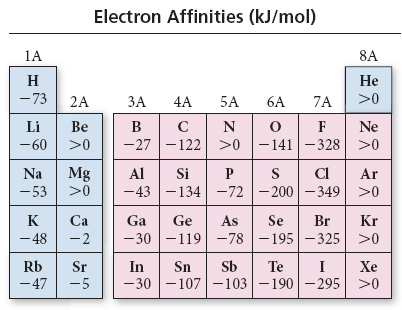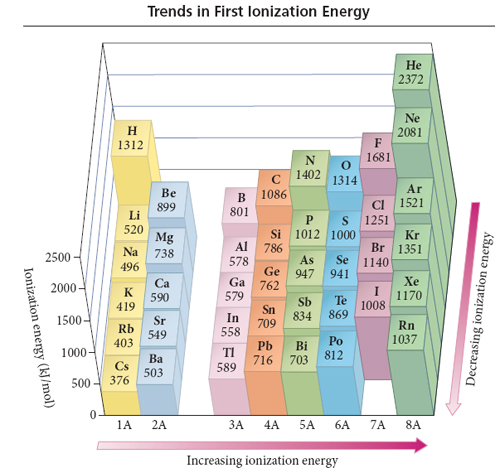# Problem: Using the data in the figures above calculate delta E for the reaction Na(g)+ Cl(g) →  Na+(g)+ Cl-(g) in kJ/mol

###### FREE Expert Solution

Recall that:

• Ionization energy →  energy in losing e-
• Electron affinity → energy in gaining e-

Na(g) →  Na+(g) + 1e-  → First ionization energy, IE

Cl(g) +  1e- Cl-(g)    → electron affinity, EA

Na(g) + Cl(g  Na+(g) + Cl-(g)                ΔE = IE + EA

$\overline{){\mathbf{∆}}{\mathbf{E}}{\mathbf{=}}{\mathbf{IE}}{\mathbf{+}}{\mathbf{EA}}}$

94% (243 ratings)###### Problem DetailsUsing the data in the figures above calculate delta E for the reaction Na(g)+ Cl(g) →  Na+(g)+ Cl-(g) in kJ/mol